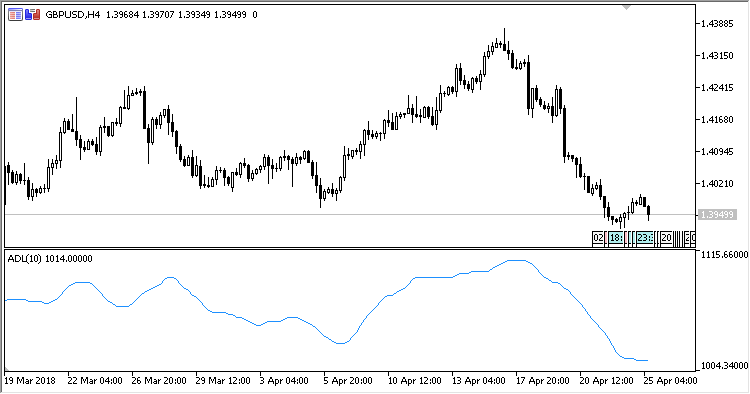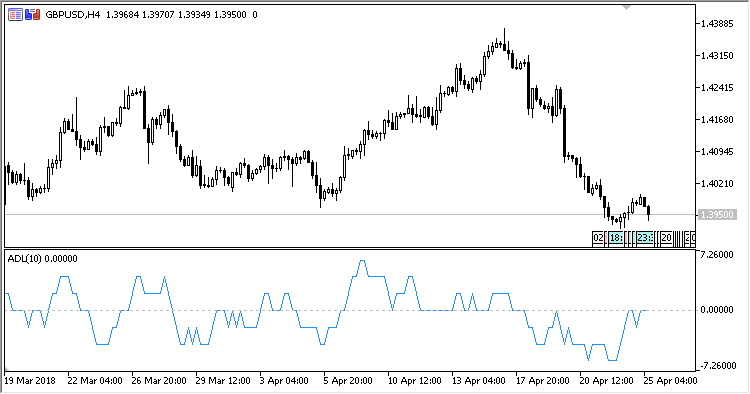# Advance_Decline_Line – indicator for MetaTrader 5

• A+
category：MT5 INDICATORS

Indicator Advance Decline Line shows the trend strength line. It can also detect possible changes in its direction.

It has two input parameters:

• Period - calculation period;
• Cumulative - calculation algorithm using the accumulated values over the Period.

Two components of the candlesticks over the period of time, i.e., the positive one and the negative one, are used for calculations. The resulting value is the difference between these two components. When using the accumulative algorithm, the indicator value calculated on the preceding bar is added to the result.Fig.1. ADL with the accumulative calculation algorithmFig.2. ADL without the accumulative calculation algorithm